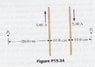# Find the direction and magnitude of the net magnetic field

cy19861126

## Homework Statement

The two wires shown below carry currents of 5.00 A in opposite directions and are separated by 10.0 cm. Find the direction and magnitude of the net magnetic field a) at a point midway between the wires b) at point P1, 10.0 cm to the right of the wire on the right, and c) at point P2, 20.0 cm to the left of the wire on the left.

B = uI/2(pi)r

## The Attempt at a Solution

I got an answer for this, but I'm not sure because my instructor has never gone over vector addition in 3D nor in magnetism. Based on what I learned before, this is what I got
a) 0. because both magnetic field are at the same magnitude but opposite direction. Therefore, their magnitudes cancel and the resultant is 0

b) B = 1.26*10^-6*5.00/(2*3.14*0.1) = 1*10^-5T
B = 1.26*10^-6*5.00/(2*3.16*0.2) = 5*10^-6T
Bnet = 1*10^-5T - 5*10^-6T = 5*10^-6T out of the page
c) B = 1.26*10^-6*5.00/(2*3.14*0.2) = 5*10^-6T
B = 1.26*10^-6*5.00/(2*3.14*0.3) = 3.33*10^-6T
Bnet = 5*10^-6 - 3.3*10^-6 = 1.7*10^-6T out of page

#### Attachments

•#34.JPG
40.4 KB · Views: 907
Last edited: